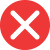grammatically

Correct spelling, explanation: grammatically is the correct form, because the root here is noun grammar, which is spelled with double m. Then, the adjective grammatical was created and then adverb grammarically, by adding suffix -ly. Therefore gramatically is an incorrect spelling.

Definition of grammatically:
adverb, in a way that relates to grammar
Sorry but this sentence is completely grammatically incorrect.
Grammatically speaking the text was okay, but it was too long and quite boring.gramatically

Incorrect spelling, explanation: this form is incorrect since the core word grammar is spelled with double m (the origins go back to Latin grammatica). Therefore gramatically should also have double m: grammatically. Grammatically is the correct form, because it is an adverb made by adding suffix -ly to the adjective grammatical.grammaticaly

Incorrect spellinggramaticaly

Incorrect spellinggramatticaly

Incorrect spelling